# Best Numerical Analysis books for Engineers & Scientists

Numerical analysis, a part of mathematics and computer science that analyzes, implements the algorithm for solving the problems of mathematical analysis. This article is very helpful for those who are interested in numerical analysis. This best books will be also great references for professionals and researchers. Anyone can learn numerical methods step by step by reading these best books.

## 1. Applied Numerical Methods W/MATLAB: for Engineers & Scientists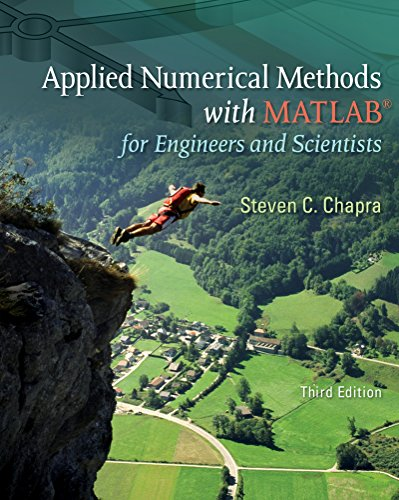Author: Steven C. Chapra Dr.
Published at: 27/01/2011
ISBN: 0073401102

Applied Numerical Methods with MATLAB for Engineering and Science is written by Steven C. Chapra who is known as best selling author. This book provides comprehensive coverage of numerical problem solving and focuses on problem-solving rather than theory, using MATLAB. The theory is included only to inform key concepts which is very helpful for Numerical Methods users. This text is written for undergraduate students taking a one-semester course in numerical analysis.

What you will learn:
•    Modeling, Computers, and Error Analysis.
•    Gauss elimination.
•    Roots and Optimization.
•    Ordinary Differential Equations.
•    Linear Systems.
•    Curve Fitting.
•    Integration and Differentiation.
•    MATLAB Built-in Functions.
•    MATLAB M-File Functions.

## 2. Numerical Analysis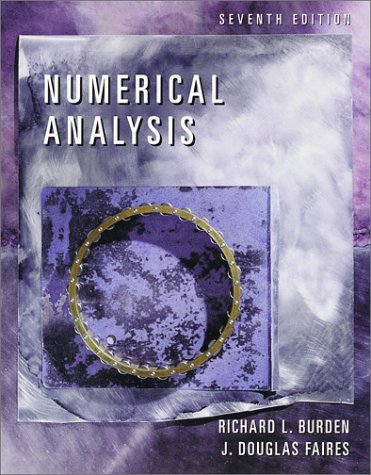Author: Richard L. Burden,J. Douglas Faires
Published at: 29/12/2000
ISBN: 0534382169

Burden and Faires’ Numerical Analysis, Seventh Edition is an excellent book that helps the students to enter the world of Numerical Analysis smoothly. The material in the book itself is a great resource, and I would argue that any CS student (or even just a programmer who wants to be a bit better in his field) should know this material and in that way, they know how to evaluate the run-time performance of a program (if nothing else). The book is fairly well understood even if you aren't the best in Math, so don't let that stop you if you are interested, there are online code snippets and evaluation programs you can try out and learn from also. The applications chosen in this edition bring out concisely how numerical methods can be applied in real-life situations.

What you will learn:
•    Mathematical  Preliminaries & Error Analysis.
•    Solutions of Equations in One Variable.
•    Interpolation and Polynomial Approximation.
•    Numerical Differentiation and Integration.
•    InitialValue Problems for Ordinary Differential Equations.
•    Direct Methods for Solving Linear Systems.
•    Iterative Techniques in Matrix Algebra.
•    Approximation Theory.
•    Approximating Eigenvalues.
•    Numerical Solutions of Nonlinear Systems of Equations.
•    BoundaryValue Problems for Ordinary Differential Equations.
•    Numerical Solutions to Partial Differential Equations
•    Bibliography

## 3. Numerical Analysis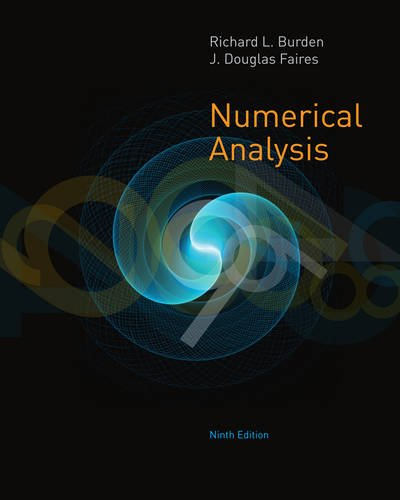Author: Richard L. Burden,J. Douglas Faires
Published at: 09/08/2010
ISBN: 0538733519

The Ninth Edition of Burden and  Faires'  Numerical Analysis is well designed for undergraduates taking one or two semesters in numerical analysis. The authors explain in which situation approximation techniques work and in which situation they fail. This edition makes the book even more helpful to the instructor and much more interesting to the reader. This book is a fantastic reference for anyone with a real mathematical background and also in a research field requiring data analysis. In this edition, one of the major changes demonstrates in Numerical Linear Algebra which has been extensively expanded.

What you will learn:
•    Mathematical  Preliminaries & Error Analysis.
•    Solutions of Equations in One Variable.
•    Interpolation and Polynomial Approximation.
•    Numerical Differentiation and Integration.
•    Initial-Value Problems for Ordinary Differential Equations.
•    Direct Methods for Solving Linear Systems.
•    Iterative Techniques in Matrix Algebra.
•    Approximation Theory.
•    Approximating Eigenvalues.
•    Numerical Solutions of Nonlinear Systems of Equations.
•    Boundary-Value Problems for Ordinary Differential Equations.
•    Numerical Solutions to Partial Differential Equations.

## 4. Numerical Methods for Scientists and Engineers (Dover Books on Mathematics)

Hamming is known as the recognized expert in coding and many fields of mathematics. The book is divided into two parts. The first one covers classical numerical methods, using classical error. The second one reexamines these methods under the frequency domain, analyzing how numerical methods affect various frequencies. This book is able to teach what other books fail to do. Many real-world examples are given and the content is well written.

What you will learn:
•    Basics of numerical methods are extremely well written.
•    Focuses on the aspects of the algorithm and its mathematical properties.
•    Difference equations and summation formulas.
•    Polynomial Approximation.
•    Fourier Approximation.
•    Digital filters and quantization.
•    Orthogonal Polynomials.
•    Discrete Fourier transforms and the FFT.
•    Linear independence.
•    Eigenvalues and eigenvectors of Hermitian matrices.

## 5. Numerical Methods for Engineers and Scientists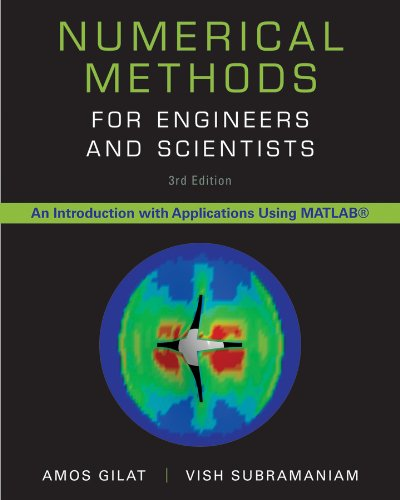Author: Amos Gilat,Vish Subramaniam
Published at: 14/10/2013
ISBN: 1118554930

Gilat and Subramaniam’s  Numerical Methods for  Engineers and Scientists, the third edition is a clear and concise guide to numerical methods for engineers and scientists. Focuses on MATLAB environment for programming practice to solve realistic problems in engineering and science. In this book, all the programs use anonymous functions. This text includes many examples and at the end of the chapter, there are three levels of homework problems which helps the students learning numerical methods. Attractive applications motivate the students.

What you will  learn:
•    Ordinary Differential Equations: Boundary-Value.
•    Ordinary Differential Equations: Initial-Value.
•    Numerical Integration.
•    Numerical Differentiation.
•    Fourier Methods.
•    Curve Fitting and Interpolation.
•    Eigenvalues and Eigenvectors.
•    Solving a System of Linear Equations.
•    Solving Nonlinear Equations.
•    Gives clear concepts of the mathematical background.

## 6. Numerical Methods for Engineers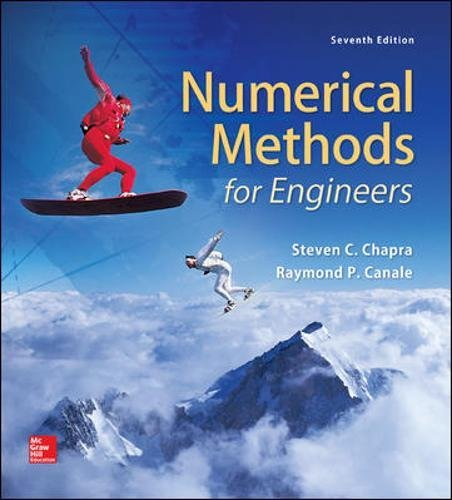Author: Steven C. Chapra Dr.,Raymond P. Canale
Published at: 24/01/2014
ISBN: 007339792X

Chapra and Canale’s Numerical Methods for Engineers, Seventh Edition is a unique approach which retains the industrial techniques that have made the book so successful. In this Seventh Edition,  an optional connect is available which is the great learning system that increases students interest to learn something new. There are multi-step-solutions of giving problems which is very helpful for students if they face any difficulty. the spreadsheets are incredibly informative than many other books.

What you will learn:
•    Modeling, Computers, and Error Analysis.
•    Roots of Equations like Bracketing Methods,  Open Methods,  Roots of Polynomials, Case Studies: Roots of Equations.
•    Linear Algebraic Equations like  Gauss Elimination, LU Decomposition and Matrix Inversion, Special Matrices and Gauss-Seidel, Case Studies: Linear Algebraic Equations.
•    Optimization.
•    Curve Fitting.
•    Numerical Differentiation and Integration.
•    Ordinary Differential Equations.
•    Partial Differential Equations.

## 7. Numerical Methods for Engineers, Sixth Edition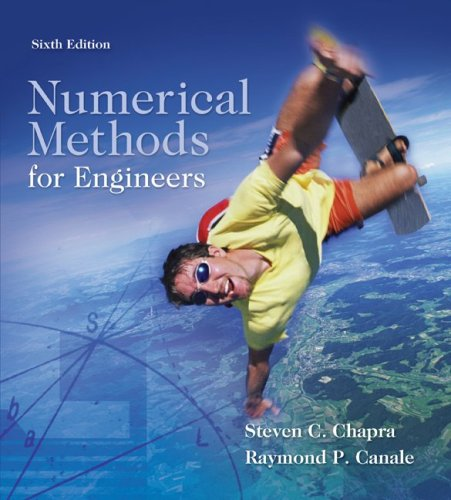Author: Steven Chapra,Raymond Canale
Published at: 20/04/2009
ISBN: 0073401064

Numerical Methods for Engineers, Sixth Edition is written by Chapra and Canale is an excellent book for understanding numerical methods easily. There are many wonderful examples using MATLAB and these are very helpful to clear both mathematical and programming concepts. Engineering students find this book much more interesting than many another book on numerical analysis. This text is a great reference for engineering students.

What you will learn:
•    Modeling, Computers, and Error Analysis.
•    Roots of Equations like Bracketing Methods,  Open Methods,  Roots of Polynomials, Case Studies: Roots of Equations
•    Linear Algebraic Equations like  Gauss Elimination, LU Decomposition and Matrix Inversion, Special Matrices and Gauss-Seidel, Case Studies: Linear Algebraic Equations
•    Optimization.
•    Curve Fitting.
•    Numerical Differentiation and Integration.
•    Ordinary Differential Equations.
•    Partial Differential Equations.

## 8. Numerical Analysis: Mathematics of Scientific Computing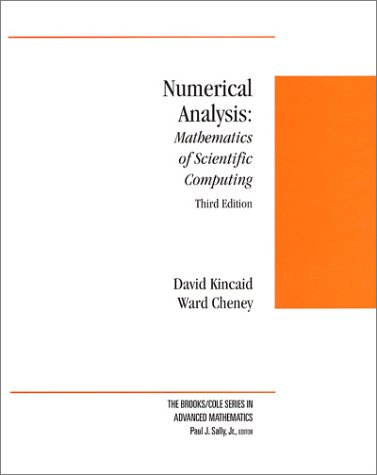Author: David R. Kincaid,E. Ward Cheney
Published at: 25/10/2001
ISBN: 0534389058

Numerical Analysis: Mathematics of Scientific Computing is written by David Kincaid and Ward Cheney for a one-semester course in numerical methods. This book treats numerical analysis from the mathematical point of view. This book will be a great reference for undergraduates.If you are a Matlab lover, the algorithms are very useful for you. Algorithms are presented in pseudocode so that students can directly write the codes from pseudocode into Matlab.

What you will learn:
•    Computer Arithmetic.
•    A solution of Nonlinear Equations.
•    Solving Systems of Linear Equations.
•    Selected Topics in Numerical Linear Algebra.
•    Approximating Functions.
•    Numerical Differentiation and Integration.
•    Numerical Solution of Ordinary.
•    Numerical Solution of Partial Differential Equations.
•    Linear Programming and Related Topics.
•    Optimization.

## 9. Analysis of Numerical Methods (Dover Books on Mathematics)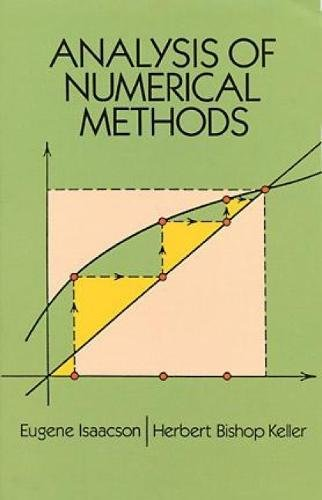Author: Eugene Isaacson,Herbert Bishop Keller
Published at: 07/06/1994
ISBN: 0486680290
Analysis of Numerical Methods (Dover Books on Mathematics) is a classic book required for any type of computational work of numerical methods. In this book, there are many practical examples, problems and their solutions are given in many cases which expand the analysis. The author focuses on Functional Analysis which is very important in the more sophisticated study on numerical methods. This text is very informative to both applied and theoretical fields of matrix algebra and bridges between linear algebra and statistical models.

What you will learn:
•    Norms, Arithmetic, and Well-Posed Computations.
•    Numerical Solution of Linear Systems and Matric Inversion.
•    Iterative Solutions and Non-Linear Equations.
•    Computation of Eigenvalues and Eigenvectors.
•    Basic Theory of Polynomial Approximation.
•    Differences, Interpolation Polynomials, and Approximate Differentiation.
•    Numerical Integration.
•    Numerical Solution of Ordinary Differential  Equations.
•    Difference Methods for Partial Differential Equations.

## 10. Numerical Analysis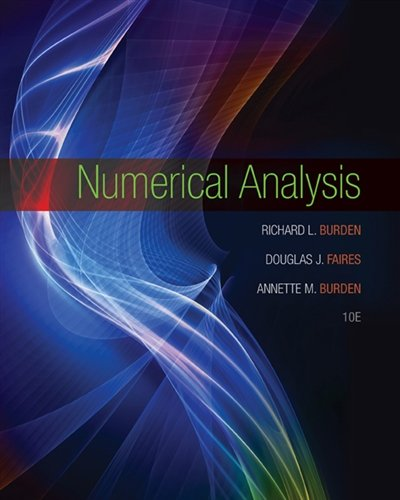Author: Richard L. Burden,J. Douglas Faires,Annette M. Burden
Published at: 01/01/2015
ISBN: 1305253663

Burden, Faires and Burden's Numerical Analysis is written for those who love to learn numerical problem solving rather than theory. In this edition, there are complete mathematical explanations and modern approximation techniques are introduced. C, Fortran, Maple,  Mathematica, MATLAB, and Pascal program are given for each algorithm. The number of the section has been expanded, some divided to make the book more useful for the readers. This book is a standard material itself and many practical applications are demonstrated so readers can understand how numerical methods are used in real-life situations.

What you will learn:
•    Mathematical  Preliminaries & Error Analysis.
•    Solutions of Equations in One Variable.
•    Interpolation and Polynomial Approximation.
•    Numerical Differentiation and Integration.
•    Initial-Value Problems for Ordinary Differential Equations.
•    Direct Methods for Solving Linear Systems.
•    Iterative Techniques in Matrix Algebra.
•    Approximation Theory.
•    Approximating Eigenvalues.
•    Numerical Solutions of Nonlinear Systems of Equations.
•    Boundary-Value Problems for Ordinary Differential Equations.
•    Numerical Solutions to Partial Differential Equations.

## 11. Numerical Methods: Design, Analysis, and Computer Implementation of Algorithms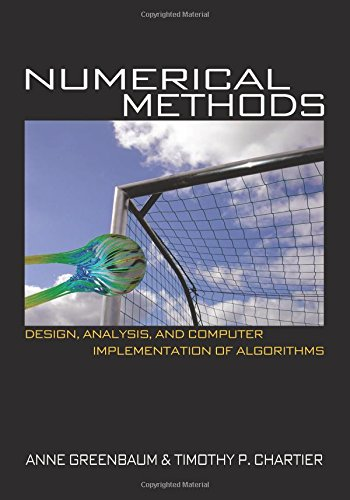Author: Anne Greenbaum,Timothy P. Chartier
Published at: 01/04/2012
ISBN: 0691151229

Numerical Methods provides a clear and concise exploration of standard numerical analysis topics, as well as nontraditional ones, including mathematical modeling, Monte Carlo methods, Markov chains, and fractals. The book also includes polynomial interpolation at Chebyshev points, use of the MATLAB package Chebfun, and a section on the fast Fourier transform. Supplementary materials are available online.

What You Will Learn

• Clear and concise exposition of standard numerical analysis topics
• Explores nontraditional topics, such as mathematical modeling and Monte Carlo methods
• Covers modern applications, including information retrieval and animation, and classical applications from physics and engineering
• Promotes understanding of computational results through MATLAB exercises
• Provides flexibility so instructors can emphasize mathematical or applied/computational aspects of numerical methods or a combination
• Includes recent results on polynomial interpolation at Chebyshev points and use of the MATLAB package Chebfun
• Short discussions of the history of numerical methods interspersed throughout
• Supplementary materials available online.

## 12. Numerical Methods in Engineering with Python 3

This book is an introduction to numerical methods for students in engineering. It covers the usual topics found in an engineering course: solution of equations, interpolation and data fitting, solution of differential equations, eigenvalue problems, and optimization. The algorithms are implemented in Python 3, a high-level programming language that rivals MATLAB® in readability and ease of use.

All methods include programs showing how the computer code is utilized in the solution of problems. The book is based on Numerical Methods in Engineering with Python, which used Python 2. This new text demonstrates the use of Python 3 and includes an introduction to the Python plotting package Matplotlib. This comprehensive book is enhanced by the addition of numerous examples and problems throughout.

Also you can check these books:
• Schaum's Outline of Numerical Analysis

• Thanks for reading this post. If you have any opinion don't hesitate to comment here. Also please subscribe our newsletter to get more updates.